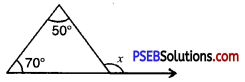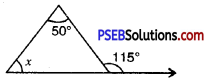# PSEB 7th Class Maths MCQ Chapter 6 Triangles Integers

Punjab State Board PSEB 7th Class Maths Book Solutions Chapter 6 Triangles Integers MCQ Questions with Answers.

## PSEB 7th Class Maths Chapter 6 Triangles Integers MCQ Questions

Multiple Choice Questions :

Question 1.
The closed curve made up of three lines segments is called :
(b) Triangle
(c) Rectangle
(d) Square
(b) Triangle

Question 2.
The number of median a triangle has, is:
(a) Two
(b) One
(c) Three
(d) Four
(c) Three

Question 3.
What is the number of altitudes of a triangle ?
(a) Three
(b) Four
(c) One
(d) Two
(a) Three

Question 4.
In the following fig. value of exterior angle x is :(a) 50°
(b) 70°
(c) 120°
(d) 60°
(c) 120°

Question 5.
In the following fig. the value of unknown interior angle x is :(a) 50°
(b) 115°
(c) 65°
(d) 130°
(c) 65°Fill in the blanks :

Question 1.
A triangle can have ……………. medians.
Three

Question 2.
An exterior angle of a triangle is equal to ……………. of its interior opposite angles.
Sum

Question 3.
A triangle is a ……………. figure.
Closed

Question 4.
Sum of the angles of a triangle is …………….
180°

Question 5.
The point of concurrence of the medians of a triangle is called …………….
CentroidWrite True or False :

Question 1.
A triangle can have three altitudes. (True/False)
True

Question 2.
There are three angle bisectors in a triangle. (True/False)
True

Question 3.
A triangle is possible with angle 60°, 70°, 80°. (True/False)